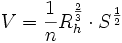The Open Channel Flow Calculator is used to determine open-channel flow in circular pipes based on Manning's Equation:where:
V is the cross-sectional average velocity (m/s)
n is the Manning coefficient of roughness
Rh is the hydraulic radius (m)
S is the slope of the water surface or the linear hydraulic head loss (m/m) (S = hf / L)